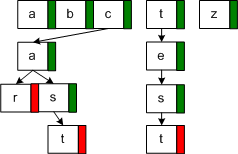## Implementing a Trie in C#

Download Trie.zip Trie is a tree like data structure that can be used for fast text search. While there are a lot of examples on the internet, very few if any are written in C#. In this post, I would like to show a complete implementation in C#. In my implementation, the Trie nodes are represented by an array of pointers. While it is not the most efficient way to do, it nevertheless gets the point through. An alternative and more efficient way to do would utilize a linked list in place of the array of pointers. The following figure illustrates how I implement the Trie structure.Here is the code for the TrieNode class.

class TrieNode {

public TrieNode[] nodes;

public bool isEnd = false;

public const int ASCIIA = 97;

public TrieNode() {

nodes = new TrieNode;

}

public bool Contains(char c) {

int n = Convert.ToByte(c) ASCIIA;

if (n < 26)

return (nodes[n] != null);

else

return false;

}

public TrieNode GetChild(char c) {

int n = Convert.ToByte(c) ASCIIA;

return nodes[n];

}

}

Whenever the TrieNode gets initialized, an array (representing 26 letters) of pointers is created. And Contains() method returns true if the corresponding node contains a reference (think of pointers) to a child. And finally, GetChild() function returns a child tree that is rooted at the character passed in. Note that the GetChild function only works level by level, e.g. for word “boot”, you will need to pass in the whole word and then scanning from left to right, and at each letter, you would call GetChild to get a tree that representing the current tree from that letter on. This makes intuitive sense, because if you do not call this function sequentially, how would you tell which “o” you are referring to? Take a look at the Tries class, and this concept should become clearer.

class Tries {

private TrieNode root = new TrieNode();

public TrieNode Insert(string s) {

char[] charArray = s.ToLower().ToCharArray();

TrieNode node = root;

foreach (char c in charArray) {

node = Insert(c, node);

}

node.isEnd = true;

return root;

}

private TrieNode Insert(char c, TrieNode node) {

if (node.Contains(c)) return node.GetChild(c);

else {

int n = Convert.ToByte(c) TrieNode.ASCIIA;

TrieNode t = new TrieNode();

node.nodes[n] = t;

return t;

}

}

public bool Contains(string s) {

char[] charArray = s.ToLower().ToCharArray();

TrieNode node = root;

bool contains = true;

foreach (char c in charArray) {

node = Contains(c, node);

if (node == null) {

contains = false;

break;

}

}

if ((node == null) || (!node.isEnd))

contains = false;

return contains;

}

private TrieNode Contains(char c, TrieNode node) {

if (node.Contains(c)) {

return node.GetChild(c);

} else {

return null;

}

}

}

Now let’s see how we would use our Trie class to check whether a given string is present from an input string.

class Program {

static void Main(string[] args) {

Tries tries = new Tries();

TrieNode n;

string s = @"

In computer science, a trie, or prefix tree,

is an ordered tree data structure that is used to

store an associative array where the keys are strings.

Unlike a binary search tree, no node in the tree

stores the key associated with that node;

instead, its position in the tree shows what key

it is associated with. All the descendants

of any one node have a common prefix of the string

associated with that node, and the root is associated

with the empty string. Values are normally not associated

with every node, only with leaves and some inner nodes

that happen to correspond to keys of interest.

";

s = s.Replace("\r\n", "");

string[] ay = s.Split(‘ ‘, ‘,’, ‘;’, ‘.’);

foreach (string str in ay) {

if (str != "")

n = tries.Insert(str);

}

Console.WriteLine(tries.Contains("prefix"));

Console.WriteLine(tries.Contains("come"));

}

}

1.ms says:

Is there any way to look for more than one word, with common letters in the same node position? For example, if the user gives “tr**”, it will return “trie” and “tree”.

2.kris says:

If I were to store both “Read” and “Reader” then how will the tree look like.

Root->R->E->A->D(IsEnd = True)
Root->R->A->D->E->R(IsEnd = true)

Is this not duplication ? Also as we have only 26 nodes under each node, there is no scope for duplication also and we cannot have the below scenario:
Root->R->E->A->D(isEnd = True)->E->R(IsEnd = True)

Then how will the 2 words “Read” and “Reader” will ge stored in the tree ?

3.Cuthahoha says:

Kris,

I ran across this looking for ways to build the Trie. I suspect in the past couple of years the answer as come, but I figured I would post and answer if anyone else comes across the same thing.

The use of the bool value “IsEnd” is unfortunate as it would lead to a misconception. It would be better to use “IsWord”, or “EndsWord”. The value is there to indicate the transversed keys constitute a word, NOT the end of the nodes in the trie. In your example the keys might look more like this.

4.Omar says: